## Thursday, September 1, 2011

### Recommendation Engine

In a classical model of recommendation system, there are "users" and "items". User has associated metadata (or content) such as age, gender, race and other demographic information. Items also has its metadata such as text description, price, weight ... etc. On top of that, there are interaction (or transaction) between user and items, such as userA download/purchase movieB, userX give a rating 5 to productY ... etc.Now given all the metadata of user and item, as well as their interaction over time, can we answer the following questions ...
1. What is the probability that userX purchase itemY ?
2. What rating will userX give to itemY ?
3. What is the top k unseen items that should be recommended to userX ?
Content-based Approach
In this approach, we make use of the metadata to categorize user and item and then match them at the category level. One example is to recommend jobs to candidates, we can do a IR/text search to match the user's resume with the job descriptions. Another example is to recommend an item that is "similar" to the one that the user has purchased. Similarity is measured according to the item's metadata and various distance function can be used. The goal is to find k nearest neighbors of the item we know the user likes.

Collaborative Filtering Approach
In this approach, we look purely at the interactions between user and item, and use that to perform our recommendation. The interaction data can be represented as a matrix.Notice that each cell represents the interaction between user and item. For example, the cell can contain the rating that user gives to the item (in the case the cell is a numeric value), or the cell can be just a binary value indicating whether the interaction between user and item has happened. (e.g. a "1" if userX has purchased itemY, and "0" otherwise.

The matrix is also extremely sparse, meaning that most of the cells are unfilled. We need to be careful about how we treat these unfilled cells, there are 2 common ways ...
• Treat these unknown cells as "0". Make them equivalent to user giving a rate "0". This may or may not be a good idea depends on your application scenarios.
• Guess what the missing value should be. For example, to guess what userX will rate itemA given we know his has rate on itemB, we can look at all users (or those who is in the same age group of userX) who has rate both itemA and itemB, then compute an average rating from them. Use the average rating of itemA and itemB to interpolate userX's rating on itemA given his rating on itemB.
User-based Collaboration Filter In this model, we do the following
1. Find a group of users that is “similar” to user X
2. Find all movies liked by this group that hasn’t been seen by user X
3. Rank these movies and recommend to user X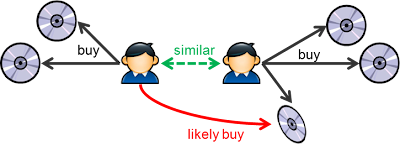This introduces the concept of user-to-user similarity, which is basically the similarity between 2 row vectors of the user/item matrix. To compute the K nearest neighbor of a particular users. A naive implementation is to compute the "similarity" for all other users and pick the top K.

Different similarity functions can be used. Jaccard distance function is defined as the number of intersections of movies that both users has seen divided by the number of union of movies they both seen. Pearson similarity is first normalizing the user's rating and then compute the cosine distance.

There are two problems with this approach
1. Compare userX and userY is expensive as they have millions of attributes
2. Find top k similar users to userX require computing all pairs of userX and userY
Location Sensitive Hashing and Minhash
To resolve problem 1, we approximate the similarity using a cheap estimation function, called minhash. The idea is to find a hash function h() such that the probability of h(userX) = h(userY) is proportion to the similarity of userX and userY. And if we can find 100 of h() function, we can just count the number of such function where h(userX) = h(userY) to determine how similar userX is to userY. The idea is depicted as follows ...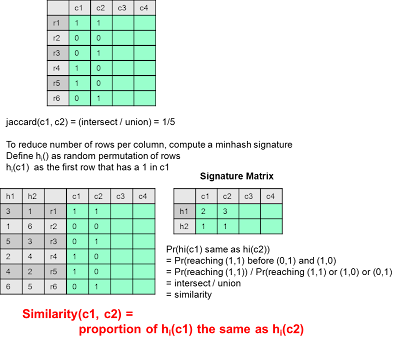Notice the picking of h() depends on the picking of the distance function.  Our distance function above is based on Jaccard distance = 1 - (Intersect/Union), and our h() is minhash.  In case a difference distance function is used (e.g. cosine distance, euclidean distance), a different h() should be picked.

Also, computing a permutation of a large number of rows can be very expensive. Remember that the purpose of h(c1) is to return row number of the first row that is 1. So we can just scan each row of c1 to see if it is 1, if it is not, we can just ignore the row number as it can never be the minimum permuted row having 1.  But if the row of c1 is 1, then there is a chance that such a row being the minimum row under the permutation.  To simulate the permutation, we scan each row and apply a function newRowNum = hash(rowNum). Take the minimum of the newRowNum seen so far.

The algorithm is as followsNotice that hash() is different from h(), which is the minhash function.  Here we use hash() just to simulate the permutation.  For each h(), we pick two random number a, b.  And we define hash(x) as the universal hash function to be ((a.x + b)%p)%N where p is a prime number much bigger than N and N is 2^32.

To solve problem 2, we need to avoid computing all other users' similarity to userX. The idea is to hash users into buckets such that similar users will be fall into the same bucket. Therefore, instead of computing all users, we only compute the similarity of those users who is in the same bucket of userX.

The idea is to horizontally partition the column into b bands, each with r rows. By pick the parameter b and r, we can control the likelihood (function of similarity) that they will fall into the same bucket in at least one band.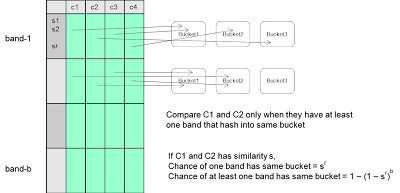Item-based Collaboration Filter
If we transpose the user/item matrix and do the same thing, we can compute the item to item similarity. In this model, we do the following ...
1. Find the set of movies that user X likes (from interaction data)
2. Find a group of movies that is similar to these set of movies that we know user X likes
3. Rank these movies and recommend to user X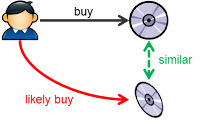It turns out that computing item-based collaboration filter has more benefit than computing user to user similarity for the following reasons ...
• Number of items typically smaller than number of users
• While user's taste will change over time and hence the similarity matrix need to be updated more frequent, item to item similarity tends to be more stable and requires less update.
Singular Value Decomposition
The user to item matrix can be considered in dual form.  Each user is represented by a vector of items and each item is represented a vector of users.

Based on SVD, every matrix can be decomposed into 3 matrices.  Therefore we can decompose the user/item Matrix into 3 matrices.  This decomposition can be interpreted as there is a hidden (latent) space between users and items (called this the concept space).  The first one U can be considered as the User/Concept matrix, The diagonal matrix Σ can be considered as the scaling of concepts (according to the strength of each concept).  The V matrix can be considered as the Item/Concept matrix.

Therefore the user / item rating should be equivalent to the cosine similarity between a user vector and item vector in the concept space.

Notice that Σ can be thought as the strength of each "concept" in the concept space. And the value is order in terms of their magnitude in decreasing order. If we remove some of the weakest concept by making them zero, we reduce the number of non-zero elements in Σ, which effective generalize the concept space (make them focus in the important concepts).

Calculate SVD decomposition for matrix with large dimensions is expensive. Fortunately, if our goal is to compute an SVD approximation (with k diagonal non-zero value), we can use the random projection mechanism as describer here.

How to compute the user vector in the concept space ?
We can use a common space (ie: the item space) between user and concept, and then project the user along each concept vector.

One challenge of determining user similarity based on item space if that if the two users are viewing different set of movies without any overlapping, then they are not considered to be similar at all (even though the movies they view are similar in the concept space).  Therefore, instead of computing the cosine similarity of two user vectors in the item space, we can transform both user vector into the concept space and compute the cosine similarity there.

When a new user arrives, how do we predict his rating on all existing items ?
Basically, we just need to compute the cosine similarity between user vector and each item vector in the concept space.

Association Rule Based
In this model, we use the market/basket association rule algorithm to discover rule like ...
{item1, item2} => {item3, item4, item5}

We represent each user as a basket and each viewing as an item (notice that we ignore the rating and use a binary value). After that we use association rule mining algorithm to detect frequent item set and the association rules. Then for each user, we match the user's previous viewing items to the set of rules to determine what other movies should we recommend.

Evaluate the recommender
After we have a recommender, how do we evaluate the performance of it ?

The basic idea is to use separate the data into the training set and the test set. For the test set, we remove certain user-to-movies interaction (change certain cells from 1 to 0) and pretending the user hasn't seen the item. Then we use the training set to train a recommender and then fit the test set (with removed interaction) to the recommender. The performance is measured by how much overlap between the recommended items with the one that we have removed. In other words, a good recommender should be able to recover the set of items that we have removed from the test set.

Leverage tagging information on items
In some cases, items has explicit tags associated with them (we can considered the tags is a user-annotated concept space added to the items). Consider each item is described with a vector of tags. Now user can also be auto-tagged based on the items they have interacted. For example, if userX purchase itemY which is tagged with Z1, and Z2. Then user will increase her tag Z1 and Z2 in her existing tag vector. We can use a time decay mechanism to update the user's tag vector as follows ...

current_user_tag = alpha * item_tag + (1 - alpha) * prev_user_tag

To recommend an item to the user, we simply need to calculate the top k items by computing the dot product (ie: cosine distance) of the user tag vector and the item tag vector.

## Sunday, August 28, 2011

### Scale Independently in the Cloud

Deploying a large scale system nowadays is quite different from before when data center is the only choice. A traditional deployment exercise typically involve a intensive performance modeling exercise to accurately predict the resource requirement for the production system. The accuracy is very important because it is expensive and slow to make changes after deploy.

This performance modeling typically involve the following steps.
1. Build a graph model based on the component interaction.
2. Express the mathematical relationship between input traffic, the resource consumption at the processing node (CPU and Memory based on the processing algorithm), and the output traffic (which will become the input of downstream processing nodes)
3. Model external workload as random variable (with a workload distribution function)
4. Run a simulation exercise to compute the corresponding workload distribution function for the workload of each link and node, such workload unit includes CPU, Memory and Network requirement (latency and bandwidth).
5. Based on business requirement, pick a peak external load target (say 95%). Vary the external workload from 0 to the max workload and compute the corresponding range of workload at each node and link in the graph.
6. The max CPU, Memory, I/O of each node defines capacity needed to provision for that node. The max value of each link defines the network bandwidth / latency requirement of that link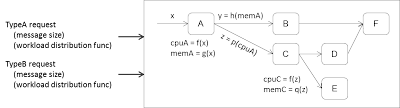Notice that the resource are typically provisioned at the peak load target which means the resources are idle most of the time, impacting the efficiency of the overall system. On the other hand, SaaS based system introduce a more dynamic relationship (anyone can call anyone) between components which makes this tradition way of performance modeling more challenging. The performance modeling exercise need to be conducted whenever new clients or new services are introduced into the system, resulting in a non-trivial on going maintenance cost.

Thanks for the cloud computing phenomenon the underlying dynamics and economics has shifted quite significantly over the last few years and now doing capacity planning is quite different from before.

First of all, making a wrong capacity estimation is less costly when deploying additional resources are talking about minutes rather than month. Instead to attempting to construct the fully picture of the system, the cloud practices is to focus at each individual component to make sure each can "scale independently". The steps are as follows ...
1. Each component scale independently using horizontal scaling. ie: f(a.x) = a.f(x)
2. Instead of establish a formal mathematical model, just deploy the system in the cloud, adjust the input workload and measure the utilization at each node and link (e.g. AWS Cloudwatch)
3. Based on the utility measurement, define the initial deployment capacity based on average load (not peak load).
4. Use auto-scaling to adjust pool size of independent components according to runtime workload.
5. Sync workload is typically frontend by Load balancer. Async workload will be frontend by scalable queues. Output can be a callout, stored in queue, or stored in scalable storage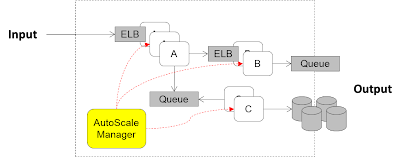By focusing in "scale independently", each component can plug and play much easier with other component due to less assumption is made on each other as each component can dynamically adjusted its capacity according to run-time need. This results in not only a more scalable, but also more flexible system.

## Saturday, July 9, 2011

### Fraud Detection Methods

Online electronic fraud has become increasingly problematic to many companies offering services on the web. Here I am trying to generalize a set of techniques that I found useful in the past.

To be effective in combating frauds, the first thing companies need to have is an overall top-down strategy to deal with frauds, including ...
1. Have a clearly defined security objective, a good understanding of the fraudsters' motivation, as well as the consequences of fraud.
2. Have an effective analytic method in place to detect fraud immediately when it happens
3. Have an responsive handling process in place to react immediately after fraud is detected
4. Have an preventive process in place to feedback newly discovered fraud patterns into the system
I will be focusing more in following discussion on the technical side of the analytic methods but I want to reiterate that the process side is equally (or even more) important in order for the whole effort of combating fraud to be effective.

Setting Objectives and Targets
Setting the objectives upfront is very important for guiding the subsequent design process of the technical mechanism, especially when making tradeoffs decisions between false positive and false negative. A high false negative rate means fraud goes through undetected while a high false positive rate will cause inconvenience to your existing customers as well as unnecessarily large manual investigation effort.

From another angle, some companies look at the fraud detection methods as an optimization mechanism of using existing resource for conducting manual investigation, which is usually the last resort to handle fraud. These companies usually has a constant team size of fraud investigators. If these people spend too much time in legitimate transactions, there will be less time left to investigate the real fraud transactions. Therefore, the analytical methods aim at guiding the manual investigation effort to those transaction with a higher chance of fraud.

Notice that fraud detection is a continuously-improvement-game. At each iteration, there is a baseline (usually the current best method) and an improvement threshold. The method at each iteration is supposed to provide at least an improvement over the baseline. In the first iteration, the baseline can be very low (e.g. simply random guess). At each iteration, the baseline will be raised until the companies' objectives and targets have been satisfactorily met.

Instrumenting Analytical Methods
Depends on the nature of business and the motivation of fraudster, the characteristics of fraud can be very different. It is very important to understand them before designing the best mechanism to combat them.

Here is a high level decision process to determine the correct methoda) Rule-base approach
If the attack pattern is well-defined (e.g. credit card fradulent transactions tend to have a higher-than-usual spending amount as well as higher-than-usual transaction rate). These attack pattern can usually be extracted from domain experts in the business. The best method in this case to implement a solution is to encode such knowledge as rules or even hard-wired into the application code for efficiency reasons.

Notice that rules need to maintain as new attack patterns are discovered or old attack patterns become obsoleted. Rule engine is a pretty common approach in order to keep such domain knowledge in a declarative form so it can be easily maintained.

b) Classification approach
If we have training examples for both normal case and fraud case, classification methods (based on machine learning) can perform very well. Such analytic methods includes logistic regression, decision trees (random forest), Support vector machine, Bayesian network (naive bayes), Neural network ... etc.

To compare the performance of different classification methods, confusion matrix is commonly used. It is a 2 by 2 matrix measuring the ratio of true positive, false positives, true negative and false negative. Based on the cost associated with false positive and false negative, we can determine a best method (or ensemble of multiple methods) to achieve a minimal cost.

c) One-Class Model approach
If we have just training examples for norm cases but no fraud examples, we still can learn a model based on normal data and then compute the distance between the transaction data and the model we learned. We flag the transaction as fraud if the distance exceed a domain-specific threshold. Here the distance function between the model and a data point needs to be defined ad commonly used ones include statistic methods where the model is the mean and standard deviation of the norm data and the P-value as the distance function. On the other hand, Euclidean distance, Jaccard distance and cosine distance are also commonly used.

d) Density based methods and clustering methods
If we know nothing about the fraud patterns and also don't have training examples for even norm cases, then we can make some assumptions about the distribution of data, such as fraud data is less dense than norm data, in other words, fraud transaction will have less neighbors within a certain radius. If this assumption is reasonable, then we can use density-based method to predict fraud transaction. For example, counting number of neighbors within radius r, or measuring the distance to the kth nearest neighbour. We can also use clustering method to learn clusters and flag transactions too distant from its cluster center as fraud.

Determining input signals
In my experience, determining the right signal is the most important part of the whole process. Sometimes we use raw input attributes as the signal while other times we need to combine multiple attributes to provide the signal.

For example, as we take raw measurements at different points in time, the input signal may involve computing the rate of change of these raw measurement over time. In other words, it is not adequate to just look at each data point in isolation and we need to aggregate raw measurement in a domain specific way.

In my past experience, a large portion of fraud detection cases is about how to deal with account takeover transactions (stolen identities and impersonation). Usually detecting sudden change of behavior (e.g. change point detection) is an effective approach to deal with this kind of frauds.

Time dimension
Instead of looking at each fraud in isolation, in many cases we need to look at the "context" under which fraud are evaluated. As we discussion above in detecting sudden change of behavior, it is quite common to use the past data of a user to build a norm model and evaluate the recent transactions against it to determine if it is fraud. In other words, we compare his/her current behavior with the past.

Besides the "time dimension", we can look into other context as well. For example, we can look at user's peer-group's behavior, observing the deviation of one person's behavior to its peer-group as an indication of a stolen identity.

Notice that the norm pattern may also evolve/change over time, nevertheless we usually don't expect such change to be sudden or rapid. To cater for such slow drift, the norm model need to be continuously adjusted as well. A pretty common technique is to compute a long-term behavioral signature based on a longer time span of transactional data (e.g. 6 months) and compute a short-term behavioral signature based on a shorter time span of data. Then the short-term signature is compared with the long-term signature using a distance function and fraud is flagged if it exceed a pre-defined threshold. It is also important to have an incremental update mechanism for the long-term signature rather than recomputing it from scratch at every update. A common approach is to use exponentially time-decay function such as ...
M[t+1] = a.M[t] + (1-a)S[t].
where 0 < a < 1
M[t] is model at time t
S[t] is the transaction at time t

The importance of Domain Experts
Although sophisticated machine learning algorithms has been pretty powerful in using a generalized solution for a broad scenarios of problems. From my past experience, I have yet seen much cases a sophisticated machine learning algorithm can beat domain expertise. In many projects, simple algorithm with deep domain expertise out-performs sophisticated analytical methods significantly. Therefore, the common pattern that I recommend is to build a rule-based solution at the core and augment it using machine learning analytical methods.

## Thursday, April 21, 2011

### K-Means Clustering in Map Reduce

Unsupervised machine learning has broad application in many e-commerce sites and one common usage is to find clusters of consumers with common behaviors. In clustering methods, K-means is the most basic and also efficient one.

K-Means clustering involve the following logical steps

1) Determine the value of k
2) Determine the initial k centroids
3) Repeat until converge
- Determine membership: Assign each point to the closest centroid
- Update centroid position: Compute new centroid position from assigned members

Determine the value of K
This is basically asking the question of: "How many clusters you are interested to discover ?"
So the answer is specific to the problem domain.

One way is to try different K. At some point, we'll see increasing K doesn't help much to improve the overall quality of clustering. Then that is the right value of K.

Notice that the overall quality of cluster is the average distance from each data point to its associated cluster.

Determine the initial K centroids
We need to pick K centroids to start the algorithm. So one way to pick them is to randomly pick K points from the whole data set.

However, picking a good set of centroids can reduce the number of subsequent iterations and by "good" I mean the K centroid should be as far apart to each other as possible, or even better the initial K centroid is close to the final K centroid. As you can see, choosing the random K points is reasonable but non-optimum.

Another approach is to take a small random sample set from the input data set and do a hierarchical clustering within this smaller set (note that hierarchical clustering is not-scaling to large data set).

We can also partition the space into overlapping region using canopy cluster technique (describe below) and pick the center of each canopy as the initial centroid.

Iteration
Each iteration is implemented as a Map/Reduce job. First of all, we need a control program on the client side to initialize the centroid positions, kickoff the iteration of Map/Reduce jobs and determine whether the iteration should end ...

``````kmeans(data) {
initial_centroids = pick(k, data)
writeToS3(initial_centroids)
old_centroids = initial_centroids
while (true){
map_reduce()
if change(new_centroids, old_centroids) < delta {
break
} else {
old_centroids = new_centroids
}
}
return result
}``````

Within each iteration, most of the processing will be done in the Map task, which determine the membership for each point, as well as compute a partial sum of each member points of each cluster.

The reducer did the easy job by aggregating all partial sums and compute the update centroid position, and then out them into a shared store (S3 in this case) that can be picked up by the Map/Reduce job of next round.Complexity Analysis
Most of the work is done by the Mapper and the workload is pretty balanced. So the time complexity will be O(k*n/p) where k is number of clusters, n is number of data points and p is number of machines. Note that the factor of k comes in at the closest_centroid() function above when comparing each data point with each intermediate centroid as follows ...
``````closest_centroid(point, listOfCentroids) {
bestCentroid = listOfCentroids
minDistance = INFINITY
for each centroid in listOfCentroids {
distance = dist(point, centroid)
if distance < minDistance {
minDistance = distance
bestCentroid = centroid
}
}
return bestCentroid
}``````

If we partition the space into proximity regions, we only need to compare each point with centroid within the same proximity region and treat other centroids infinite distance. In other words, we don't have to compare each point with all k centroids.

Canopy clustering provide such a partitioning mechanism.

Canopy Clustering
To define the proximity region (canopy), we can draw a circle (or hypersphere) centered at a data point. Points outside this sphere is considered to be too far.

However, if we apply this definition to every point, then we will have as many proximity region as the number of points, which ends up doesn't save much processing. We also observed that points are very close by each other can stay in the same region without each point creating their own. Therefore, we can draw a smaller circle within the big circle (with the same center) such that data points within the small circle is not allowed to form its own proximity region.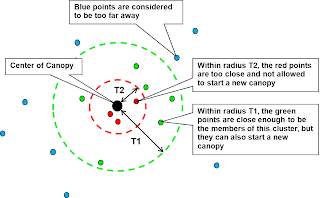Notice that each proximity region can overlap with each other and the degree of overlapping will be affected by the choice of T1. Also the choice of T2 affects how many canopies will be formed. Picking the right number of T1 and T2 is domain-specific, and also depends on the number of clusters and the space volume. If there is a small number of clusters within a big space, then a bigger T1 should be chosen.

To create the canopies (and mark the data points with the canopies), we will do the following steps ...
1) Create the canopy centers, with one scan
• Keep a list of canopies, initially an empty list
• Scan each data point, if it is within T2 distance of existing canopies, discard it. Otherwise, add this point into the list of canopies2) Assign data points to the canopies, with another scan
• Scan each data point, if it is within T1 of the canopyA, add A as the assigned canopy to the data point. Notice that the data point can be assigned to multiple canopies
• When done, each data point will look like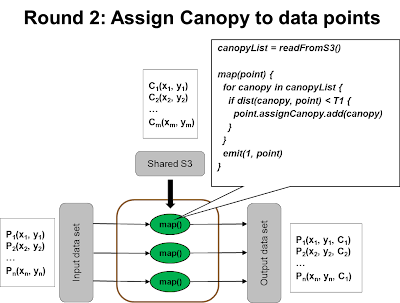Notice that now the input data points has been added with an extra attribute that contains the assigned canopies. When compare the point with the intermediate centroids, we only need to compare centroids within the same canopy. Here is the modified version of the algorithm ...

``````closest_centroid(point, listOfCentroids) {
bestCentroid = listOfCentroids
minDistance = INFINITY
for each cent in listOfCentroids {
if (not point.myCanopy.intersects(cent.myCanopy)) {
continue
}
distance = dist(point, centroid)
if distance < minDistance {
minDistance = distance
bestCentroid = centroid
}
}
return bestCentroid
}``````

## Saturday, March 19, 2011

### Compare Machine Learning models with ROC Curve

ROC Curve is a common method to compare performance between different models. It can also be used to pick trade-off decisions between "false positives" and "false negatives". ROC curve is defined as a plot of "false positive rate" against "false negative rate". However, I don't find the ROC concept is intuitive and has been struggled for a while to grasp the concept.

Here is my attempt to explain ROC curve from a different angle. We use a binary classification example to illustrate the idea. (ie: predicting whether a patient has cancer or not)

First of all, all predictive model is not 100% correct. The desirable state is that a person who actually has cancer got a positive test result, and a person who actually has no cancer got a negative test result. Since the test is imperfect, it is possible that a person who actually has cancer was tested negative (ie: Fail to detect) or a person who actually has no cancer was tested positive (ie: False alarm).In reality, there is always a tradeoff between the false negative rate and the false positive rate. People can tune the decision threshold to adjust them (e.g. In "random forest", we can set the threshold of predicting positive when more than 30% decision trees predicting positive). Usually, the threshold is set based on the consequence or cost of mis-classification. (e.g. in this example, fail to detect has a much higher cost than a false alarm)This can also be used to compare model performance. A good model is one that has both low false positive rate and low false negative rate, which is indicated in the size of the gray area below (the smaller the better).

"Random guess" is the worst prediction model and is used as a baseline for comparison. The decision threshold of a random guess is a number between 0 to 1 in order to determine between positive and negative prediction.ROC Curve is basically what I have described above with one transformation, which is transforming the y-axis from "fail to detect" to 1 - "fail to detect", which now become "success to detect". Honestly I don't understand why this representation is better though.

Now, the ROC curve will look as follows ...## Thursday, March 17, 2011

### Predictive Analytics Conference 2011

I attended the San Francisco Predictive Analytic conference this week and got a chance to chat with some best data mining practitioners of the country. Here summarizes my key takeaways.

How is the division of labor between human and machine?

Another way to ask this question is how “machine learning” and “domain expertise” work together and complement each other, since each has different strength and weakness.

Machine learning is very good at processing large amount of data in an unbiased way while human is unable to process the same data volume and the judgment is usually biased. However, machine cannot look beyond the data being given. For example, if the prediction power is low, machine learning methods cannot distinguish whether it is because the data is not clean, or the wrong model is being chosen, or because some important input feature is not captured. Domain expertise must be brought in to figure out the problem.

So the consensus is data mining / machine learning is simply a toolbox that can be used to augment human’s domain expertise, but can never replace it. For example, the domain expert can throw in a large number of input features to the machine learning model, which can determine a subset that are most influential. But if the domain expert doesn’t recognize an important input feature (and not capturing it), there is no way the machine learning model can figure out what is missing, not even recognizing that something is missing.

On the other hand, human is also very good in visualizing data patterns. “Data visualization” technique can be a powerful means to get a good sense and quickly identify the area where drilldown analysis should be conducted. Of course, visualization is limited to low dimension data as human cannot comprehend more than a handful of dimensions. Human is also easily biased so they may find patterns where are actually coincidence. By having human and machine working together, they complement each other very well.

What are some of the key design decisions in data mining?
1. Balance between false +ve and false –ve based on cost / consequence of making a wrong decision.
2. We don’t have to use a method from beginning to end. We can use different methods at different stage of the analysis. For example, in a multi-class (A, B, C) problem, we can use decision tree to distinguish A from notA (ie: B, C) and then use support vector machine to separate B and C. As another example, we can use decision tree to determine the best input attributes to be used by the neural network.

What is the most powerful / most commonly used supervised machine learning modeling technique?

The general answer is that each modeling technique has its strength and weakness and none of them wins in all situations. So understand their corresponding strength and weakness is important to pick the right one.

Generalized Linear Regression
Linear and Logistic regression are based on fitting a linear plane into a set of data points such that the root mean square of error (distance between predicted output and actual output) is minimized. It is by far the most commonly used technique, one for numeric output and the other for categorical output. They have a long history in statistics. It is supported in pretty much all commercial and open source data mining tools.

Linear and Logistic regression model requires certain amount of data preparation such as missing data handling. It also assuming that the output (or logit output) is a linear combination of input features, error is expected to be normally distribution. However, real-life scenarios are not always linear. To deal with non-linearity, input terms will be mixed (usually by cross-multiplication) in different ways to generate additional input terms called “interactions”. This process is like trial and error and can generate huge number of combination. Nevertheless, they do a reasonably good job in a wide spectrum of business problems and are well-understood by statisticians and data miners. And they are commonly used as a baseline comparison with other models.

Neural Network
Neural Network is based on multiple layer of perceptrons (each is like a logistic regression with binary input and output). There is typically a hidden layer (so the number of layers is 3) with N perceptrons (where N is trial and error). Because of the extra layer and the logit() function in the neural network, it can handle non-linearity very well. If it has good predictor in its input data, Neural network can achieve very high performance in prediction.

Similar to linear regression, Neural network requires careful data preparation to remove noisy data as well as redundant input attributes (those that are highly correlated). Neural network also take much longer time to train as compared to other methods. Also the model that Neural network has learned is not explainable or make good sense out of it.

Support Vector Machine
Support Vector Machine is a binary classifier (input feature is numeric). It is based on finding a linear plane that can separate the binary output class such that the margin is maximized. The optimal solution is expressed in terms of the dot product of vectors. If the points are not linearly separable, we can use a function to transform the points to a higher dimension space such that it is linearly separable. The Math shows that the dot product (after transforming to a hi-dim space) can be generalized into a Kernel function (Radial basis function being the most common one). Although the underlying math is not easy for everyone to understand, SVM has demonstrated outstanding performance in a wide spectrum of problems and recently become one of the most effective methods.

Despite of its powerful capability, SVM is not broadly implemented in commercial products as there are some patent issue as AT&T holds the patent of SVM. On the other hand, the non-linear kernel function (such as the most common Radial Basis function) is difficult to implement in parallel programming model such as Map/Reduce. SVM is undergoing active research and a derivative Support Vector Regression can be used to predict numeric output.

Tree Ensembles

This is combining “ensemble methods” with “decision tree”.

Decision tree is the first generation machine learning algorithm based on a greedy approach. For a classification problem, decision tree try to split a branch where the combined “purity” (either by the Gini index or Entropy) after split is maximized. For a regression problem, decision tree try to split where the combined “between-class-variance” divided by “within-class-variance” can be maximized. This is equivalent to maximizing the F-value after split. The splitting continues until reaching the terminating condition such as there are too few member remains in the branch, or the gain of further split is insignificant.

Decision tree are very good at dealing with missing value (simply not using that value in learning and go own both path in scoring). Using a decision tree to capture the decision model is also very comprehensible and explainable. However, decision tree is relatively sensitive to noise and can easily overfit the data. Although the learning mechanism is easy to understand, Decision tree doesn’t perform very well in general and is rarely used in real system. However, when decision trees are used together with Ensemble methods, it becomes extraordinary powerful as all its weakness now disappears.

The idea of ensemble is simple. Instead of learning one model, we learning multiple models and combine the estimation of each individual learner (e.g. we let them vote on categorical output and compute the average for numeric output).

There are two main models for creating different learners. One is called “bagging”, which is basically drawing samples (with replacement) from the training set and then have the same Tree algorithm to learn on different sample data set. Another model is called “boosting”, which has a sequence of iterations where samples are drawn from the training set based on the probability distribution where the wrongly predicted items in last round will have a higher chance to be selected. In other words, the algorithm places more attention to learn from wrongly-classified examples.

It turns out Ensemble tree is the most popular method at this moment as it achieve very good prediction across the board, easy to understand and can be implemented in Map/reduce. Google recently published a good paper on their PLANET project which implements ensemble tree on map/reduce.